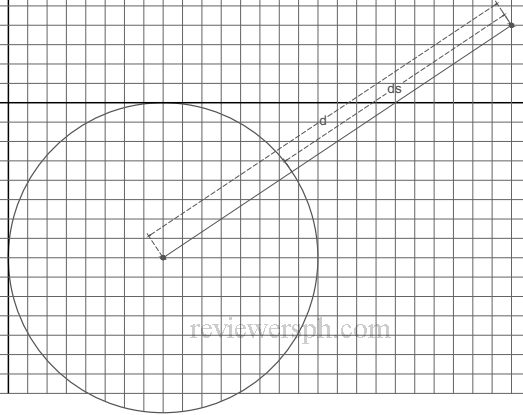### Math Notes

Subjects

#### Analytic Geometry Solutions

##### Topics || Problems

What is the shortest distance between the curve $$x^2 -16x +y^2 +16y +64 =0$$ and the point (26,4)?

Solution:

Identify the curve and locate some important points:

$$x^2 -16x +y^2 +16y = -64$$

$$x^2 -16x +64 +y^2 +16y + 64= -64 +64 +64$$

$$(x-8)^2+(y+8)^2= 8^2$$

This curve is a circle with radius = 8, and center at (8, -8)$$d = \sqrt{(26-8)^2 + (4+8)^2 }$$

$$d = 6 \sqrt{13}$$

$$d_s = 6 \sqrt{13} - 8 = 13.63$$ units